## Detailed introduction

For an extremely detailed introduction, please see

help.start()

In this documentation, the above command will be executed at the command prompt, see below.

## Brief introduction to R

From help.start():

R is an integrated suite of software facilities for data manipulation, calculation and graphical display.

RStudio is an integrated development environment (IDE) for R.

### R interface

In contrast to many other statistical software packages that use a point-and-click interface, e.g. SPSS, JMP, Stata, etc, R has a command-line interface. The command line has a command prompt, e.g. >, see below.

>

This means, that you will be entering commands on this command line and hitting enter to execute them, e.g.

help()

Use the up arrow to recover past commands.

hepl()
help() # Use up arrow and fix

### R GUI (or RStudio)

Most likely, you are using a graphical user interface (GUI) and therefore, in addition, to the command line, you also have a windowed version of R with some point-and-click options, e.g. File, Edit, and Help.

In particular, there is an editor to create a new R script. So rather than entering commands on the command line, you will write commands in a script and then send those commands to the command line using Ctrl-R (PC) or Command-Enter (Mac).

a = 1
b = 2
a+b
##  3

Multiple lines can be run in sequence by selecting them and then using Ctrl-R (PC) or Command-Enter (Mac).

### Intro Activity

One of the most effective ways to use this documentation is to cut-and-paste the commands into a script and then execute them.

Cut-and-paste the following commands into a new script and then run those commands directly from the script using Ctrl-R (PC) or Command-Enter (Mac).

x = 1:10
y = rep(c(1,2), each=5)
m = lm(y~x)
s = summary(m)

Now, look at the result of each line

x
y
m
s
s$r.squared When you have completed the activity, compare your results to the solutions. ## Using R as a calculator ### Basic calculator operations All basic calculator operations can be performed in R. 1+2 ##  3 1-2 ##  -1 1/2 ##  0.5 1*2 ##  2 For now, you can ignore the  at the beginning of the line, we’ll learn about that when we get to vectors. ### Advanced calculator operations Many advanced calculator operations are also available. (1+3)*2 + 100^2 # standard order of operations ##  10008 sin(2*pi) # the result is in scientific notation, i.e. -2.449294 x 10^-16  ##  -2.449294e-16 sqrt(4) ##  2 10^2 ##  100 log(10) # the default is base e ##  2.302585 log(10, base=10) ##  1 ### Using variables A real advantage to using R rather than a calculator (or calculator app) is the ability to store quantities using variables. a = 1 b = 2 a+b ##  3 a-b ##  -1 a/b ##  0.5 a*b ##  2 ### Assignment operators =, <-, and -> When assigning variables values, you can also use arrows <- and -> and you will often see this in code, e.g. a <- 1 2 -> b c = 3 # is the same as <- Now print them. a ##  1 b ##  2 c ##  3 ### Using informative variable names While using variables alone is useful, it is much more useful to use informative variables names. population = 1000 number_infected = 200 deaths = 3 death_rate = deaths / number_infected attack_rate = number_infected / population death_rate ##  0.015 attack_rate ##  0.2 ### Calculator Activity #### Bayes’ Rule Suppose an individual tests positive for a disease, what is the probability the individual has the disease? Let • $$D$$ indicates the individual has the disease • $$N$$ means the individual does not have the disease • $$+$$ indicates a positive test result • $$-$$ indicates a negative test The above probability can be calculated using Bayes’ Rule: $P(D|+) = \frac{P(+|D)P(D)}{P(+|D)P(D)+P(+|N)P(N)} = \frac{P(+|D)P(D)}{P(+|D)P(D)+(1-P(-|N))\times(1-P(D))}$ where Calculate the probability the individual has the disease if the test is positive when • the specificity of the test is 0.95, • the sensitivity of the test is 0.99, and • the prevalence of the disease is 0.001. When you have completed the activity, compare your results to the solutions. ## Reading data into R In this section, we will learn how to read in csv or Excel files into R. We focus on csv files because they are simplest to import, they can be easily exported from Excel (or other software), and they are portable, i.e. they can be used in other software. ### Changing your working directory One of the first tasks after starting R is to change the working directory. To set, • in RStudio: Session > Set Working Directory > Choose Directory… (Ctrl + Shift + H) • in R GUI (Windows): File > Change Dir… • in R GUI (Mac): Misc > Change Working Directory… Or, you can just run the following command setwd(choose.dir(getwd())) Make sure you have write access to this directory. ### Installing and loading a package Much of the functionality of R is contained in packages. The first time these packages are used, they need to be installed, e.g. to install a package from CRAN use install.packages('dplyr') Once installed, a package needs to be loaded into each R session where the package is used. library('dplyr') ### Load and start this workshop First load the package library('ISDSWorkshop') This package contains a function to help you get started, so run that function. workshop() This function did three things: 1. It opened the workshop outline in a web browser. 2. It created a set of .csv data files in your working directory. 3. It created a set of .R scripts in your working directory. ### Open an R script As we progress through the workshop, the code for a particular module will be available in the R script for that module. In R/RStudio, open the module called 01_intro.R and scroll down to the workshop() command. From here on out, as I run commands you should run the commands as well by using Ctrl-R (Windows) or Command-Enter (Mac) with the appropriate line(s) highlighted. You will notice that nothing after a # will be evaluated by R. That is because the # character indicates a comment in the code. For example, # This is just a comment. 1+1 # So is this ##  2 # 1+2 ### Reading data into R Data are stored in many different formats. I will focus on data stored in a csv file, but mention approaches to reading in data stored in Excel, SAS, Stata, SPSS, and database formats. #### Reading a csv file into R The most common way I read data into R is through a csv file. csv stands for comma-separated value file and is a standard file format for data. The utils package (which is installed and loaded with base R) has a function called read.csv for reading csv files into R. For example, GI = read.csv("GI.csv") This created a data.frame object in R called GI. The utils package has the read.table() function which is a more general function for reading data into R and it has many options. We could have gotten the same results if we had used the following code: GI2 = read.table("GI.csv", header=TRUE, # There is a header. sep=",") # The column delimiter is a comma. To check if the two data sets are equal, use the following all.equal(GI, GI2) ##  TRUE The read.csv function is available in base R, but these days I will often use the read_csv function in the readr. install.packages("readr") # run this command if the readr package is not installed library('readr') GI <- read_csv("GI.csv") ### Read Excel xlsx file My main suggestion for reading Excel files into R is to 1. Save the Excel file as a csv 2. Read the csv file into R using read.csv This approach will work regardless of any changes Excel makes in its document structure. Reading an Excel xlsx file into R is done using the read.xlsx function from the xlsx R package. Unfortunately many scenarios can cause this process to not work. Thus, we do not focus on it in an introductory R course. When it works, it looks like this install.packages('xlsx') library('xlsx') d = read.xlsx("filename.xlsx", sheetIndex=1) # or d = read.xlsx("filename.xlsx", sheetName="sheetName") Again, if these approaches don’t work, you can Save as... a csv file in Excel. ### Read SAS, Stata, or SPSS data files The haven package provides functionality to read in SAS, Stata, and SPSS files. An example of reading a SAS file is install.packages('haven') library('haven') d = read_sas('filename.sas7bdat') #### Read a database file There are many different types of databases, so the code you will need will be specific to the type of database you are trying to access. The dplyr package, which we will discussing today, has a number of functions to read from some databases. The code will look something like library('dplyr') my_db <- src_sqlite("my_db.sqlite3", create = T) The RODBC package has a number of functions to read from some databases. The code might look something like install.packages("RODBC") library('RODBC') # RODBC Example # import 2 tables (Crime and Punishment) from a DBMS # into R data frames (and call them crimedat and pundat) library(RODBC) myconn <-odbcConnect("mydsn", uid="Rob", pwd="aardvark") crimedat <- sqlFetch(myconn, "Crime") pundat <- sqlQuery(myconn, "select * from Punishment") close(myconn) ### Exploring the data set There are a number of functions that will provide information about a data.frame. Here are a few: dim(GI) ##  21244 9 nrow(GI) ##  21244 ncol(GI) ##  9 names(GI) # column names ##  "id" "date" "facility" "icd9" ##  "age" "zipcode" "chief_complaint" "syndrome" ##  "gender" head(GI, n=5) # first 5 rows of the data.frame ## id date facility icd9 age zipcode chief_complaint ## 1 1001301988 2005-02-28 67 787.01 7 21075 Abd Pain ## 2 1001829757 2005-02-28 67 558.90 41 20721 upset stomach ## 3 1001581758 2005-02-28 123 787.91 2 22152 diarrhea ## 4 1001950471 2005-02-28 123 787.91 71 22060 ABD PAIN ## 5 1001076304 2005-02-28 309 558.90 28 21702 LOWER AD PAIN ## syndrome gender ## 1 GI Male ## 2 GI Female ## 3 GI Male ## 4 GI Male ## 5 GI Female tail(GI, n=5) # last5 rows of the data.frame ## id date facility icd9 age zipcode ## 21240 1001392877 2008-12-30 123 787.01 6 22153 ## 21241 1001887911 2008-12-30 37 535.50 72 22033 ## 21242 1001196061 2008-12-30 6200 536.20 59 20155 ## 21243 1001067104 2008-12-30 67 558.90 80 22202 ## 21244 1001396039 2008-12-30 123 787.03 12 20112 ## chief_complaint syndrome gender ## 21240 N/V/D GI Male ## 21241 VOMITING CHILLS GI Male ## 21242 LEFT CHEST AND ABDOMINAL PAIN GI Male ## 21243 ABD PAIN GI Female ## 21244 Diarrhea GI Male ### Activity If you brought your own Excel file, open it and save a sheet as a csv file in your working directory. If you brought your own csv file, save it in your working directory. If you did not bring your own file, use the fluTrends.csv file in your working directory. Try to use the read.csv function to read the file into R. There are a number of different options in the read.table() function that may be useful: d = read.table("filename.csv", # Make sure to change filename>to your filename and # make sure you use the extension, e.g. .csv. header = TRUE, # If there is no header column, change TRUE to FALSE. sep =",", # The column delimiter is a comma. skip = 0 # Skip this many lines before starting to read the file ) You may also need to look at the help file for read.table() to find additional options that you need. ?read.table When you have completed the activity, compare your results to the solutions. ## Descriptive statistics When reading your data set into R, you will likely want to perform some descriptive statistics. The single most useful command to assess the whole data set is the summary() command: summary(GI) ## id date facility icd9 ## Min. :1.001e+09 2007-01-31: 57 Min. : 37 Min. : 3.0 ## 1st Qu.:1.001e+09 2007-01-29: 55 1st Qu.: 66 1st Qu.: 558.9 ## Median :1.001e+09 2007-01-16: 52 Median : 67 Median : 787.0 ## Mean :1.001e+09 2007-02-28: 52 Mean :1102 Mean : 1043.2 ## 3rd Qu.:1.002e+09 2007-01-24: 50 3rd Qu.: 309 3rd Qu.: 787.3 ## Max. :1.002e+09 2007-01-17: 45 Max. :7298 Max. :78791.0 ## (Other) :20933 ## age zipcode chief_complaint syndrome ## Min. : 0.00 Min. :20001 Abd Pain : 1390 GI:21244 ## 1st Qu.: 8.00 1st Qu.:20747 ABD PAIN : 1074 ## Median : 27.00 Median :21740 Vomiting : 661 ## Mean : 29.98 Mean :21420 VOMITING : 563 ## 3rd Qu.: 47.00 3rd Qu.:22182 ABDOMINAL PAIN: 452 ## Max. :157.00 Max. :22556 vomiting : 423 ## (Other) :16681 ## gender ## Female:10653 ## Male :10591 ## ## ## ## ##  ### Descriptive statistics for continuous (numeric) variables To access a single column in the data.frame use a dollar sign ($).

GI$age # or GI[,'age'] # or GI[,5] # since age is the 5th column Here are a number of descriptive statistics for age: min(GI$age)
##  0
max(GI$age) ##  157 mean(GI$age)
##  29.98221
median(GI$age) ##  27 quantile(GI$age, c(.025,.25,.5,.75,.975))
##   2.5%    25%    50%    75%  97.5%
##  0.075  8.000 27.000 47.000 81.000
summary(GI$age) ## Min. 1st Qu. Median Mean 3rd Qu. Max. ## 0.00 8.00 27.00 29.98 47.00 157.00 Anything look odd here? ### Descriptive statistics for categorical (non-numeric) variables The table() function provides the number of observations at each level of a categorical variable. table(GI$gender)
##
## Female   Male
##  10653  10591

which is the same as summary() if the variable is not coded as numeric

summary(GI$gender) ## Female Male ## 10653 10591 If the variable is coded as numeric, but is really a categorical variable, then you can still use table, but summary won’t give you the correct result. table(GI$facility)
##
##   37   66   67  123  255  256  259  309  390  413  420  522  703 6200 6201
## 3571 2950 4281 4408   41  575    1  325  661  668  100   67  178 1423 1928
## 7298
##   67
summary(GI$facility) ## Min. 1st Qu. Median Mean 3rd Qu. Max. ## 37 66 67 1102 309 7298 Apparently there is only 1 observation from facility 259, was that a typo? ### Filtering the data Rather than having descriptive statistics for the dataset as a whole, we may be interested in descriptive statistics for a subset of the data, i.e. you want to filter() the data. The following code creates a new data.frame() that only contains observations from facility 37: library('dplyr') GI_37 <- GI %>% filter(facility == 37) # Notice the double equal sign! nrow(GI_37) # Number of rows (observations) in the new data set ##  3571 The following code creates a new data.frame that only contains observations with chief_complaint “Abd Pain”: GI_AbdPain <- GI %>% filter(chief_complaint == "Abd Pain") # Need to quote non-numeric variable level nrow(GI_AbdPain) ##  1390 #### Alternative ways to filter There are many other ways to subset/filter the data, but these days I almost exclusively use dplyr::filter() as I find the code is much easier to read. GI_37a = GI[GI$facility==37,]
all.equal(GI_37, GI_37a)
##  "Attributes: < Component \"row.names\": Mean relative difference: 4.978973 >"
GI_37b = subset(GI, facility==37)
all.equal(GI_37, GI_37b)
##  "Attributes: < Component \"row.names\": Mean relative difference: 4.978973 >"
GI_AbdPain1 = GI[GI$chief_complaint == "Abd Pain",] all.equal(GI_AbdPain, GI_AbdPain1) ##  "Attributes: < Component \"row.names\": Mean relative difference: 13.83397 >" GI_AbdPain2 = subset(GI, chief_complaint == "Abd Pain") all.equal(GI_AbdPain, GI_AbdPain2) ##  "Attributes: < Component \"row.names\": Mean relative difference: 13.83397 >" #### Advanced filtering We can subset continuous variables using other logical statements. GI %>% filter(age < 5) GI %>% filter(age >= 60) GI %>% filter(chief_complaint %in% c("Abd Pain","ABD PAIN")) # Abd Pain or ABD PAIN GI %>% filter(tolower(chief_complaint) == "abd pain") # any capitalization pattern GI %>% filter(!(facility %in% c(37,66))) # facility is NOT 37 or 66 ### Descriptive statistics on the subset Now we can calculate descriptive statistics on this subset, e.g. summary(GI_37$age)
##    Min. 1st Qu.  Median    Mean 3rd Qu.    Max.
##     3.0    19.0    36.0    39.3    58.0   139.0
summary(GI_AbdPain$age) ## Min. 1st Qu. Median Mean 3rd Qu. Max. ## 0.00 7.00 27.00 28.61 45.00 157.00 ### Activity Find the min, max, mean, and median age for zipcode 20032. When you have completed the activity, compare your results to the solutions. ## Graphical statistics Here we focus on the graphical options available in the base package graphics. • Histograms (hist()) • Boxplots (boxplot()) • Scatter plots (plot()) • Bar charts (barplot()) Although I sometimes use these base graphics, I end up switching to ggplot2 graphics very quickly. ### Histograms For continuous variables, histograms are useful for visualizing the distribution of the variable. hist(GI$age)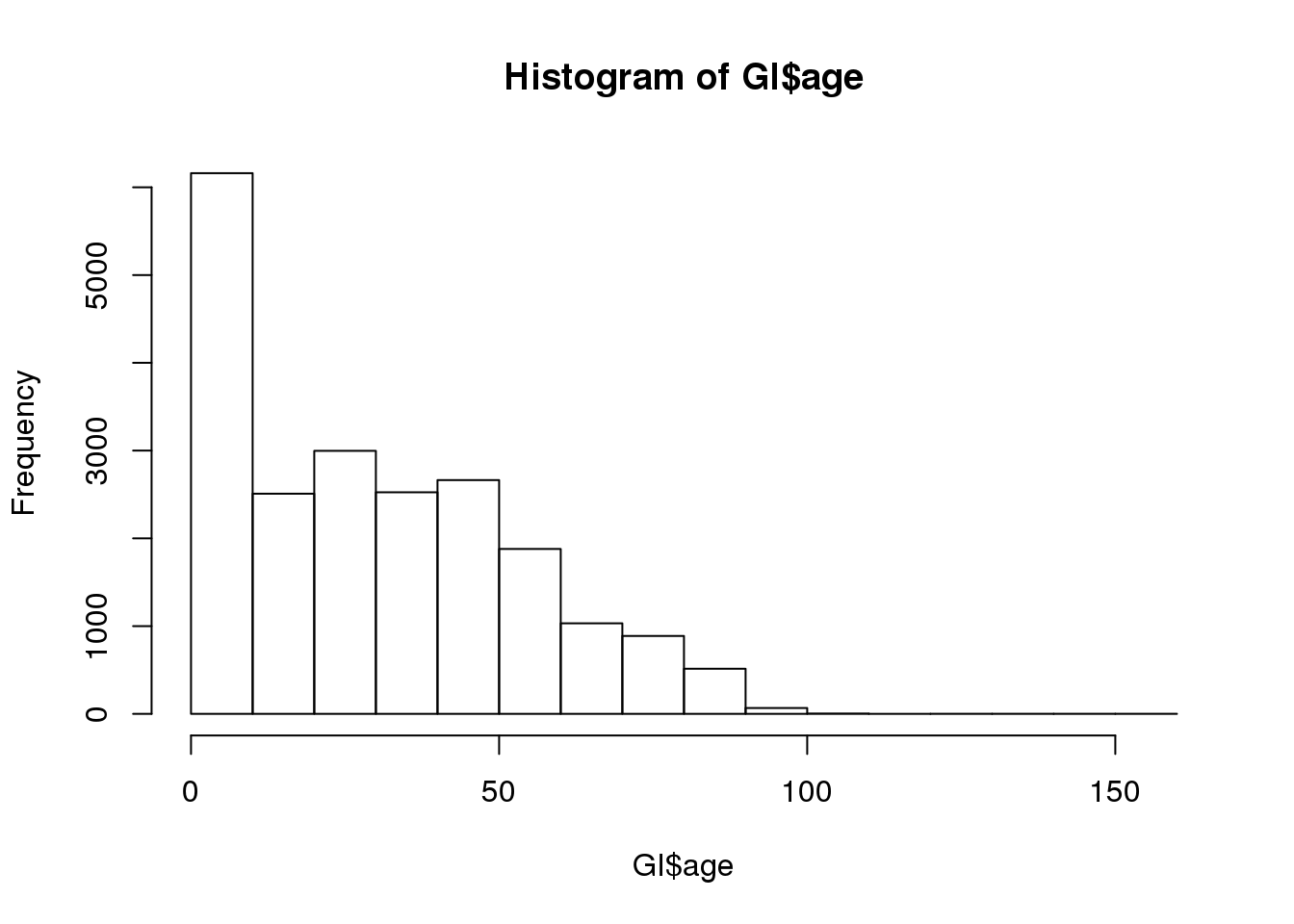When there is a lot of data, you will typically want more bins

hist(GI$age, 50)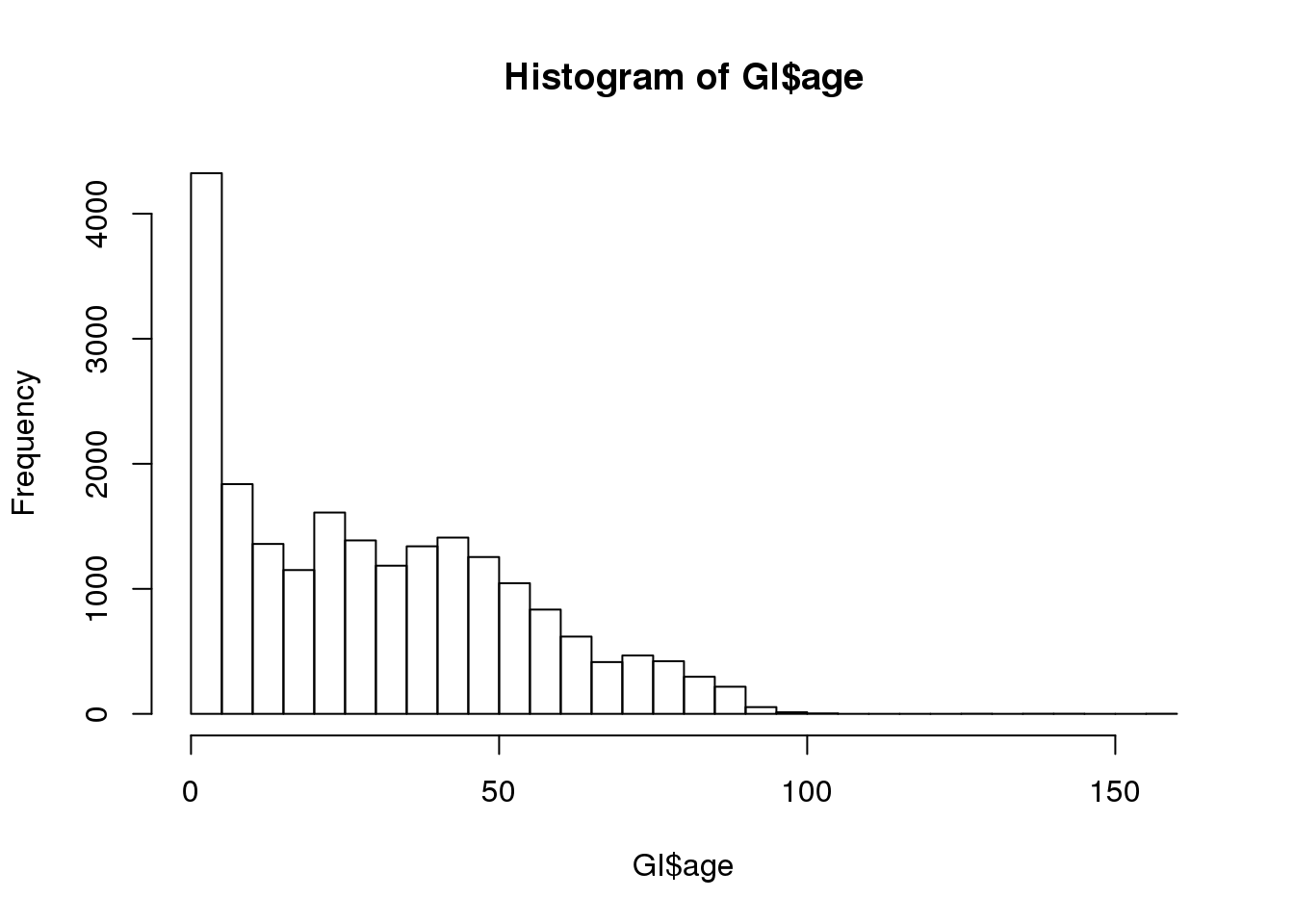You can also specify your own bins hist(GI$age, 0:158)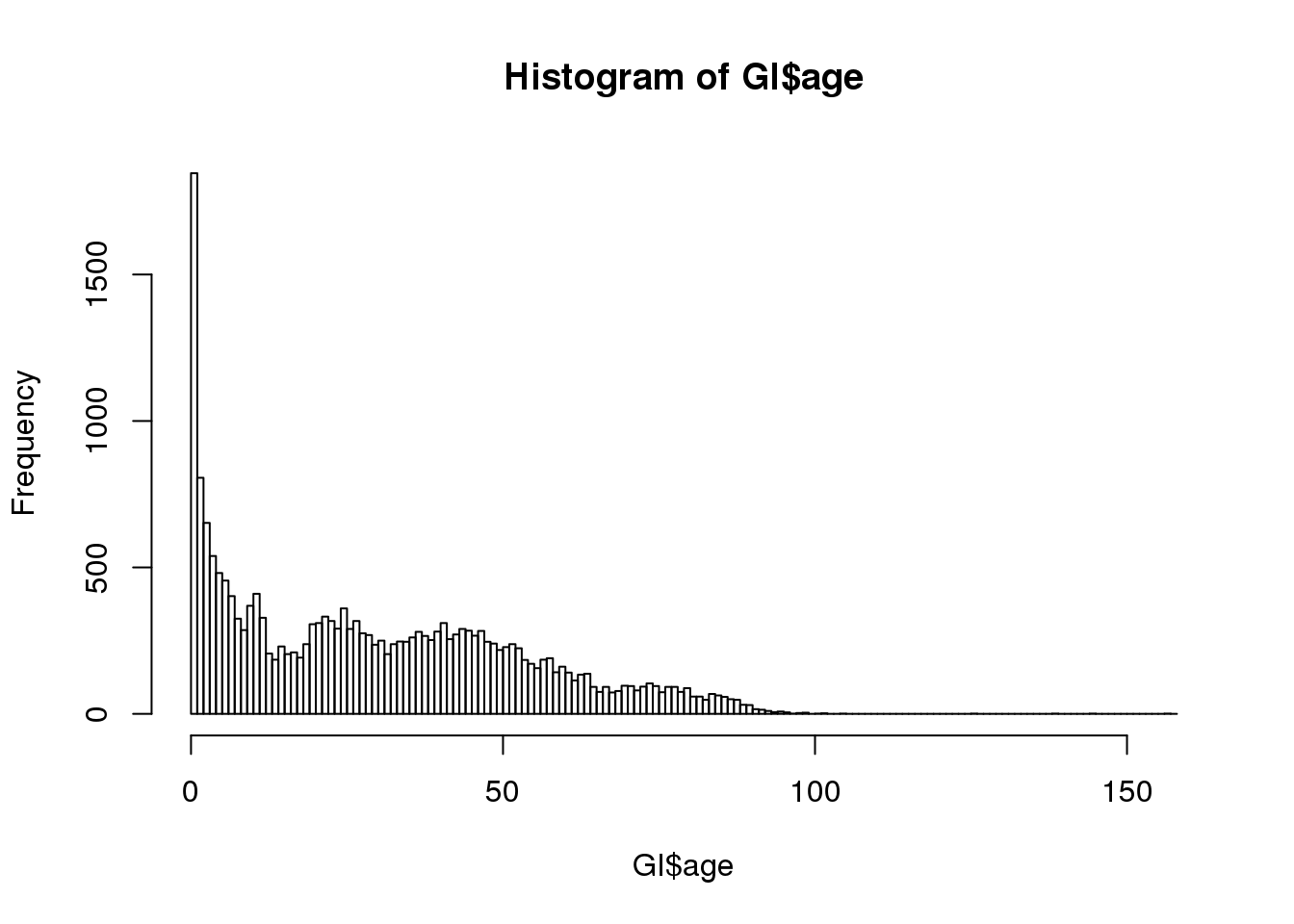### Boxplots

Boxplots are another way to visualize the distribution for continuous variables.

boxplot(GI$age)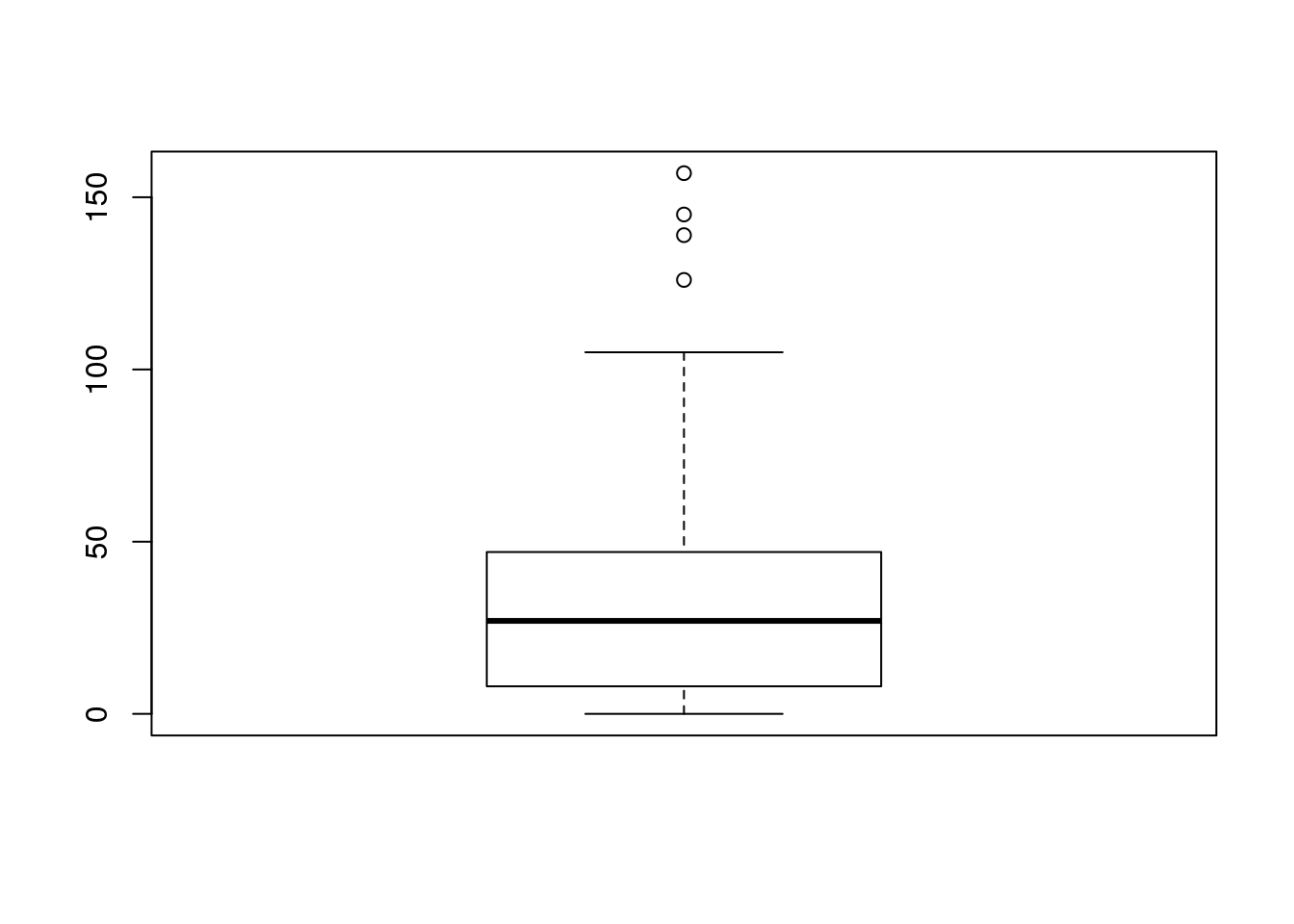Now we can see the outliers. #### Multiple boxplots Here we create separate boxplots for each facility and label the x and y axes. boxplot(age ~ facility, data = GI, xlab = "Facility", ylab = "Age")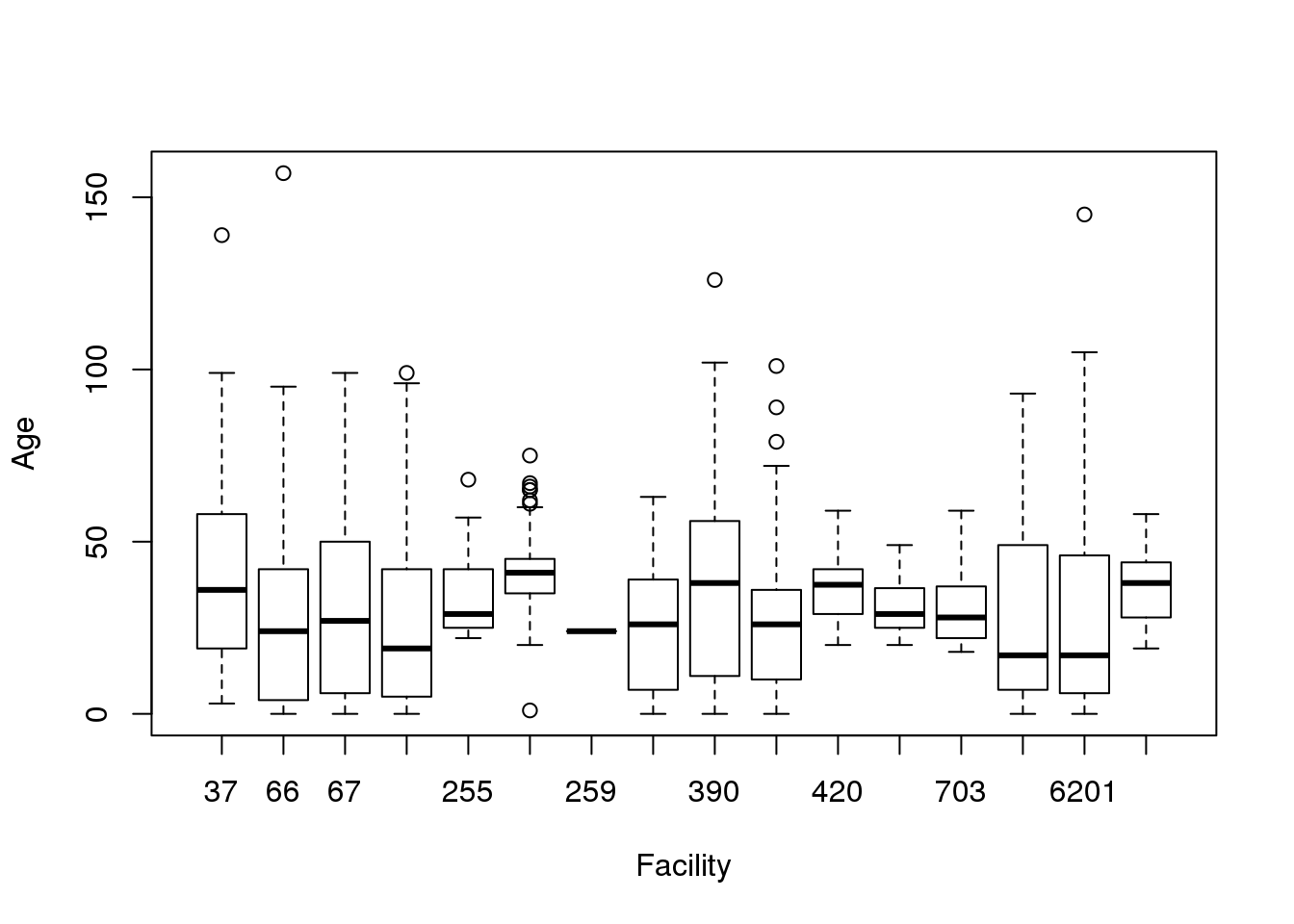### Scatterplots Scatterplots are useful for looking at the relationship of two continuous variables. GI$date = as.Date(GI$date) plot(age ~ date, data = GI)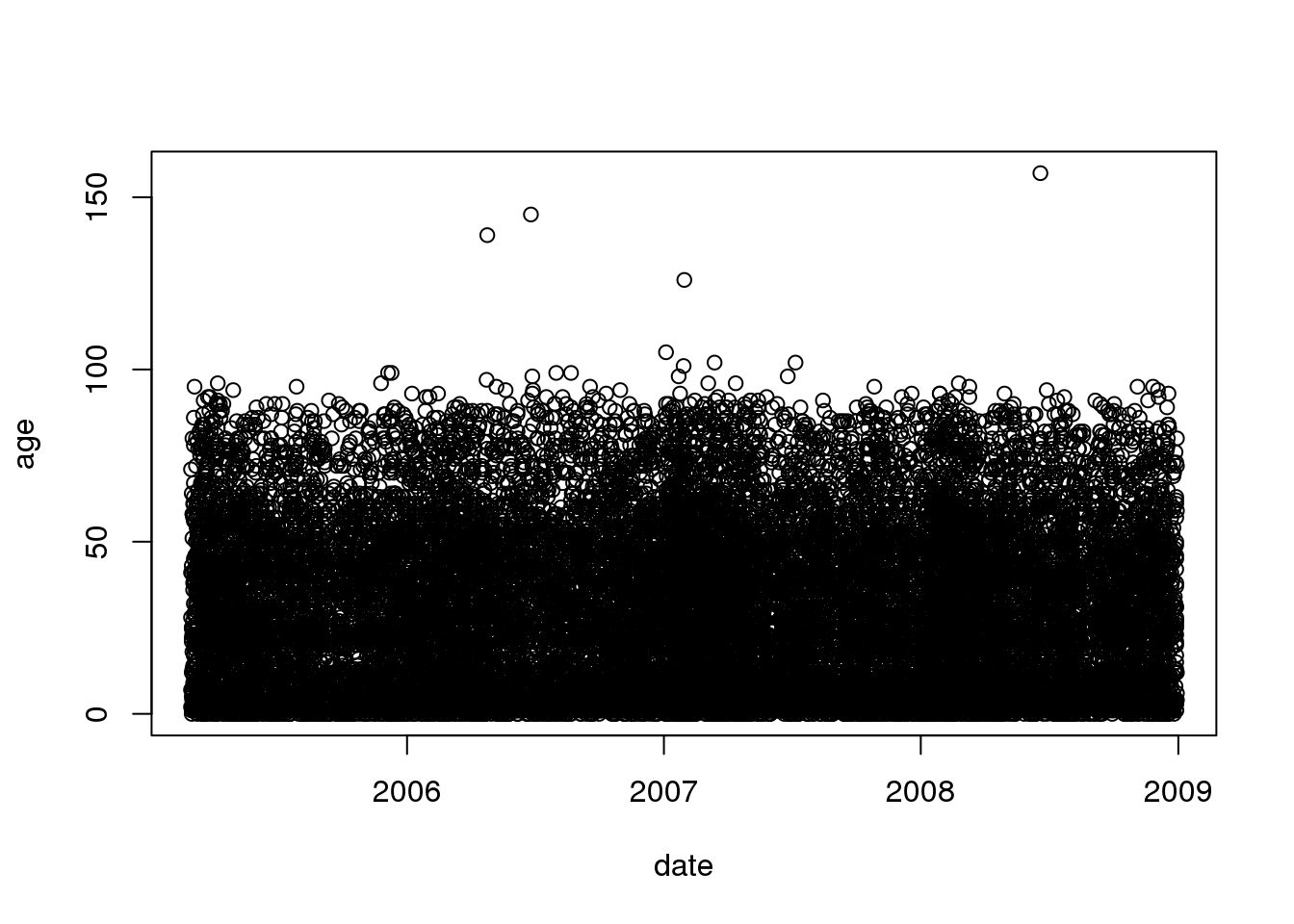We will talk more later about dealing with dates later. ### Bar charts For looking at the counts of categorical variables, we use bar charts. counts = table(GI$facility)
barplot(counts,
xlab = "Facility",
ylab = "Count",
main = "Number of observations at each facility")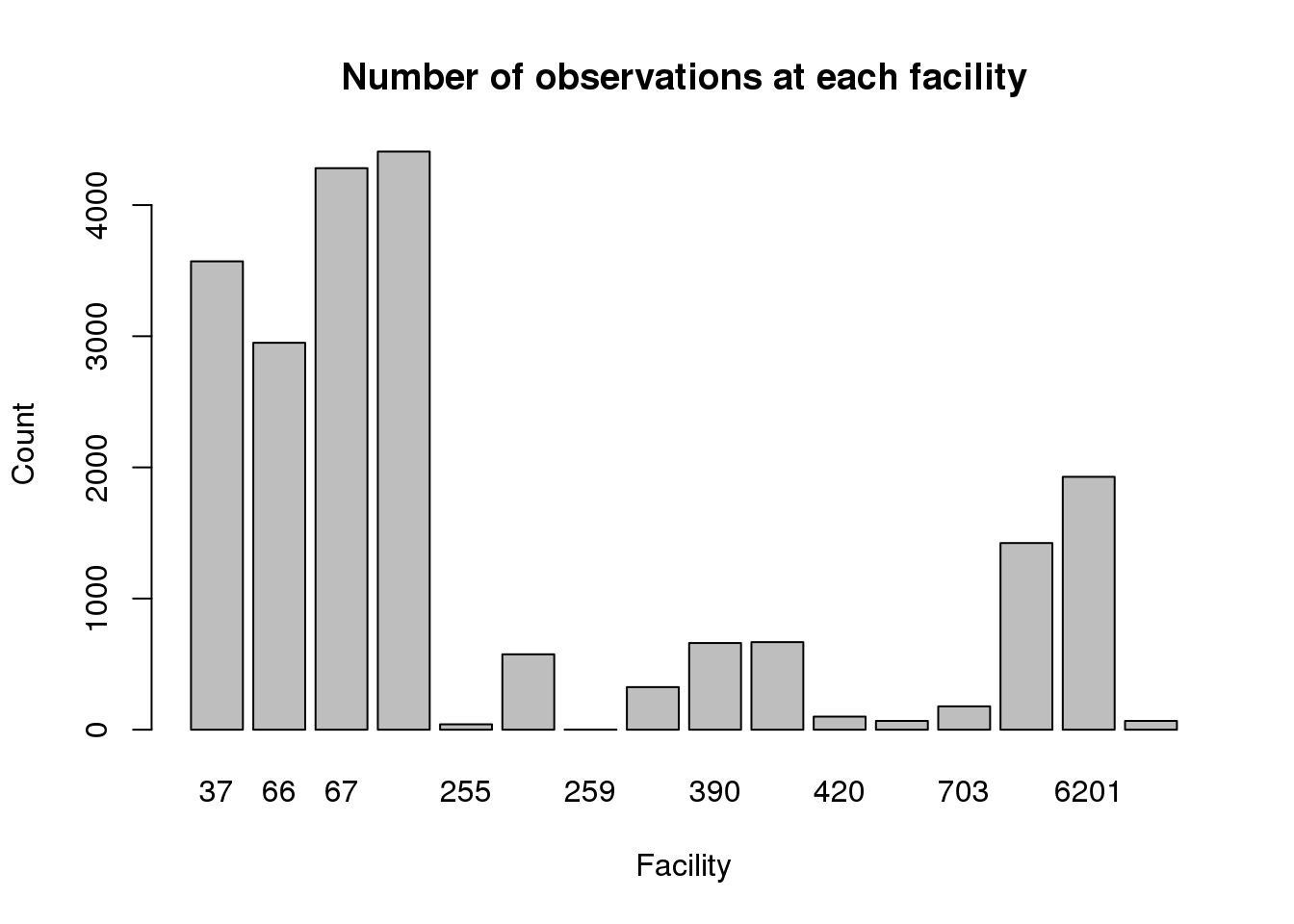### Activity

Construct a histogram and boxplot for age at facility 37.

Construct a bar chart for the zipcode at facility 37.

When you have completed the activity, compare your results to the solutions.

## Getting help

As you work with R, there will be many times when you need to get help.

My basic approach is

1. Use the help contained within R
2. Perform an internet search for an answer
3. Find somebody else who knows

In all cases, knowing the R keywords, e.g. a function name, will be extremely helpful.

### Help within R I

If you know the function name, then you can use ?<function>, e.g.

?mean

The structure of help is - Description: quick description of what the function does - Usage: the arguments, their order, and default values (if any) - Arguments: more thorough description about the arguments - Value: what the funtion returns - See Also: similar functions - Examples: examples of how to use the function

### Help within R II

If you cannot remember the function name, then you can use help.search("<something>"), e.g.

help.search("mean")

Depending on how many packages you have installed, you will find a lot or a little here.

### Internet search for R help

I google for <something> R, e.g.

calculate mean R

Some useful sites are